# Graphing Quadratic Functions Chapter 8 Graphing fx ax

• Slides: 53Graphing f(x) = ax 2 + bx + c I can graph quadratic functions of the form f(x) = ax 2 + bx + c.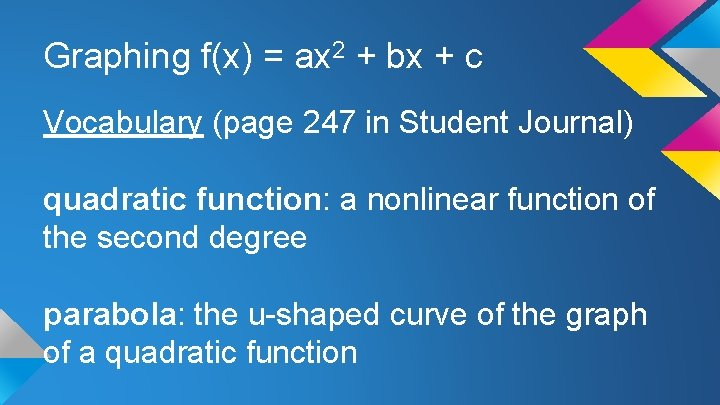Graphing f(x) = ax 2 + bx + c Vocabulary (page 247 in Student Journal) quadratic function: a nonlinear function of the second degree parabola: the u-shaped curve of the graph of a quadratic functionGraphing f(x) = ax 2 + bx + c vertex: the lowest, or highest, point on a parabola, which is on the axis of symmetry: the line that divides a parabola into 2 matching halves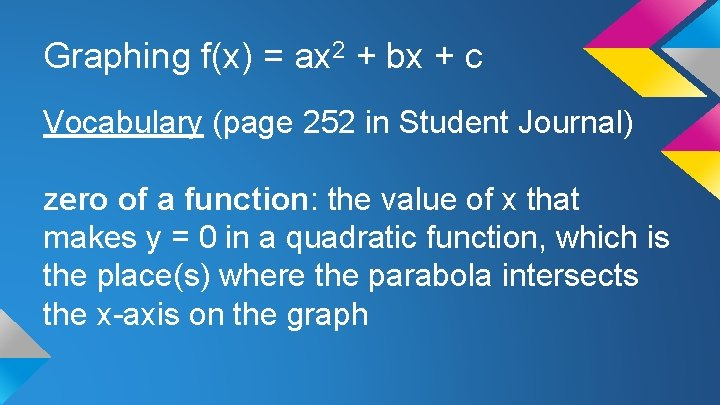Graphing f(x) = ax 2 + bx + c Vocabulary (page 252 in Student Journal) zero of a function: the value of x that makes y = 0 in a quadratic function, which is the place(s) where the parabola intersects the x-axis on the graph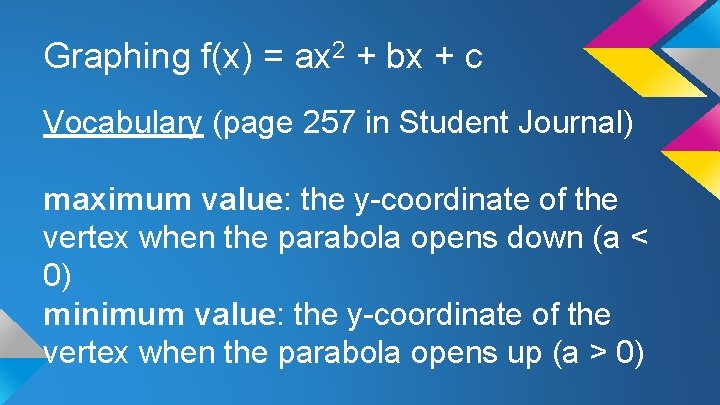Graphing f(x) = ax 2 + bx + c Vocabulary (page 257 in Student Journal) maximum value: the y-coordinate of the vertex when the parabola opens down (a < 0) minimum value: the y-coordinate of the vertex when the parabola opens up (a > 0)Graphing f(x) = ax 2 + bx + c Core Concepts (pages 247 and 248 in Student Journal) Graphing f(x) = ax 2 -when a > 1 the graph of f(x) = x 2 is a vertical stretch -when 0 < a < 1 the graph of f(x) = x 2 is a vertical shrinkGraphing f(x) = ax 2 + bx + c Graphing f(x) = ax 2 (continued) -when a < -1 the graph of f(x) = x 2 is a vertical stretch and is reflected in the x-axis -when -1 < a < 0 the graph of f(x) = x 2 is a vertical shrink and is reflected in the xaxisGraphing f(x) = ax 2 + bx + c Core Concepts (page 257 in Student Journal) Graphing f(x) = ax 2 + bx + c -when a > 0, the graph opens up -when a < 0, the graph opens down -the y-intercept is c -the x-coordinate of the vertex is –b/2 aGraphing f(x) = ax 2 + bx + cGraphing f(x) = ax 2 + bx + c Solution #1) x = 5, (5, -23)Graphing f(x) = ax 2 + bx + cGraphing f(x) = ax 2 + bx + c Solution #5)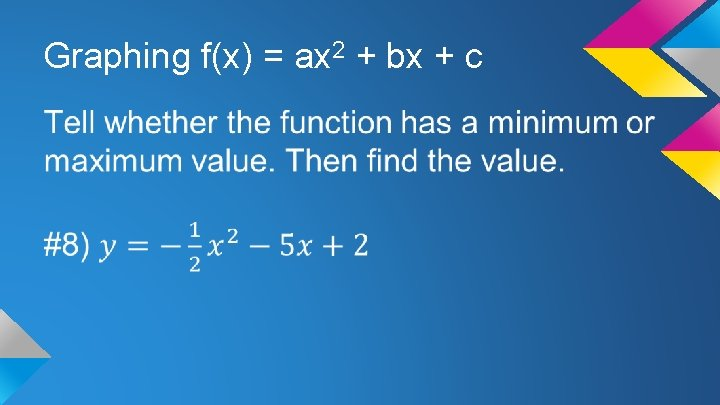Graphing f(x) = ax 2 + bx + c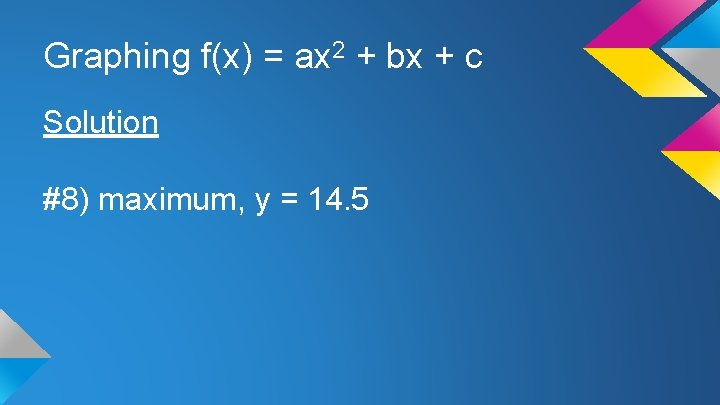Graphing f(x) = ax 2 + bx + c Solution #8) maximum, y = 14. 5Graphing f(x) = ax 2 + bx + c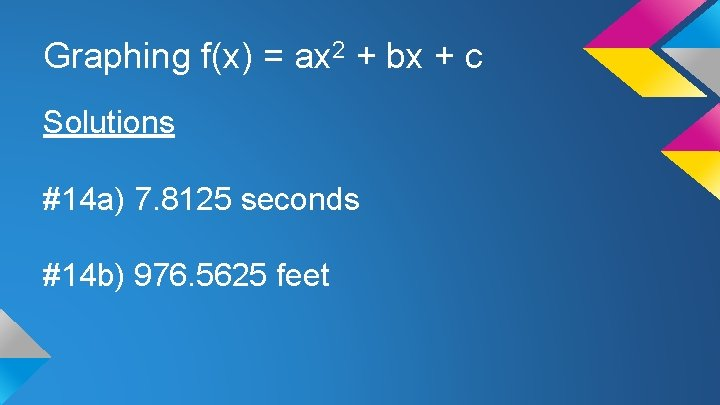Graphing f(x) = ax 2 + bx + c Solutions #14 a) 7. 8125 seconds #14 b) 976. 5625 feet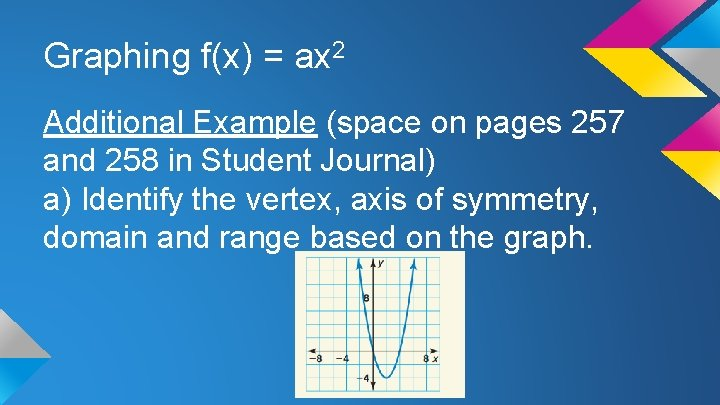Graphing f(x) = ax 2 Additional Example (space on pages 257 and 258 in Student Journal) a) Identify the vertex, axis of symmetry, domain and range based on the graph.Graphing f(x) = ax 2 Solution a) vertex at (2, -4), axis of symmetry is x = 2, domain is all real numbers, range is y greater than or equal to -4Graphing f(x) = a(x – h)2 + k I can graph quadratic functions in the form f(x) = a(x – h)2 + k.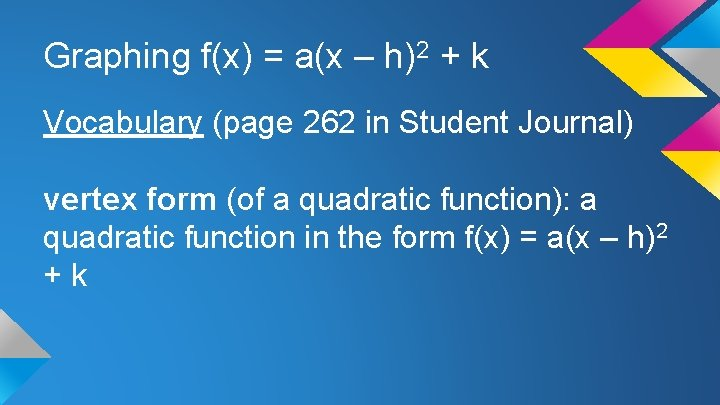Graphing f(x) = a(x – h)2 + k Vocabulary (page 262 in Student Journal) vertex form (of a quadratic function): a quadratic function in the form f(x) = a(x – h)2 + kGraphing f(x) = a(x – h)2 + k Core Concepts (pages 262 and 263 in Student Journal) Graphing f(x) = a(x – h)2 + k -a is the vertical stretch/shrink factor -h is the horizontal translation -k is the vertical translation -(h, k) are the coordinates of the vertex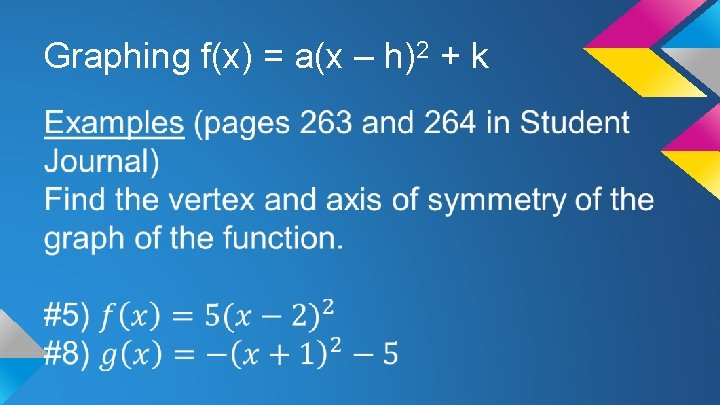Graphing f(x) = a(x – h)2 + kGraphing f(x) = a(x – h)2 + k Solutions #5) (2, 0), x = 2 #8) (-1, -5), x = -1Graphing f(x) = a(x – h)2 + k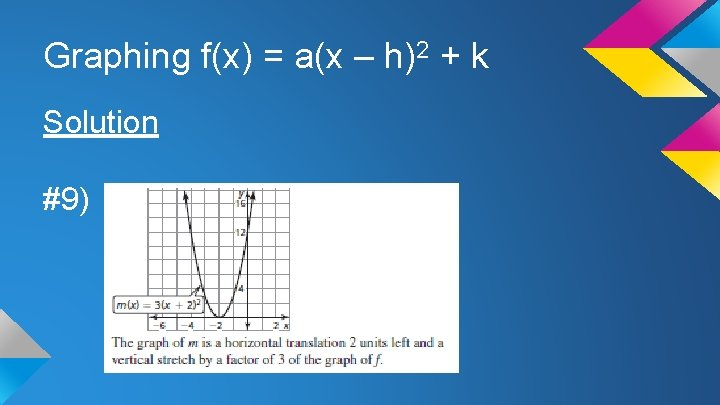Graphing f(x) = a(x – h)2 + k Solution #9)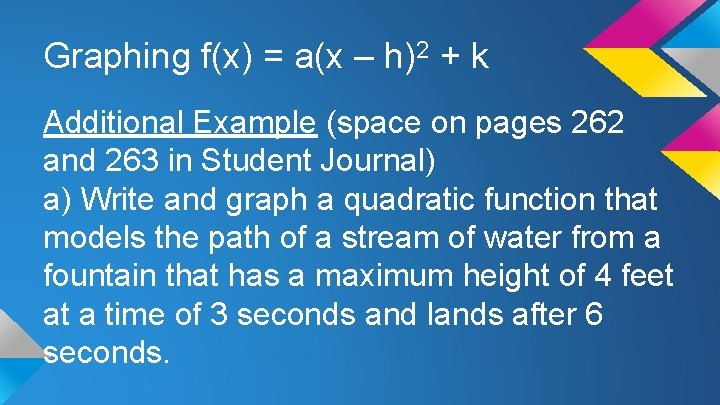Graphing f(x) = a(x – h)2 + k Additional Example (space on pages 262 and 263 in Student Journal) a) Write and graph a quadratic function that models the path of a stream of water from a fountain that has a maximum height of 4 feet at a time of 3 seconds and lands after 6 seconds.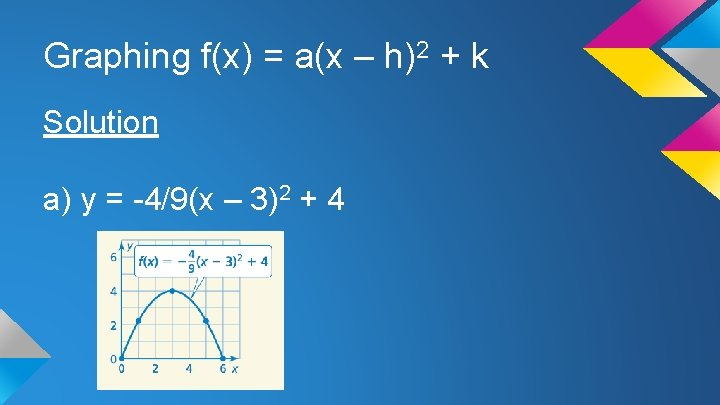Graphing f(x) = a(x – h)2 + k Solution a) y = -4/9(x – 3)2 + 4Using Intercept Form I can graph quadratic functions of the form f(x) = a(x – p)(x – q).Using Intercept Form Vocabulary (page 267 in Student Journal) intercept form: a quadratic function written in factored, which is f(x) = a(x – p)(x – q)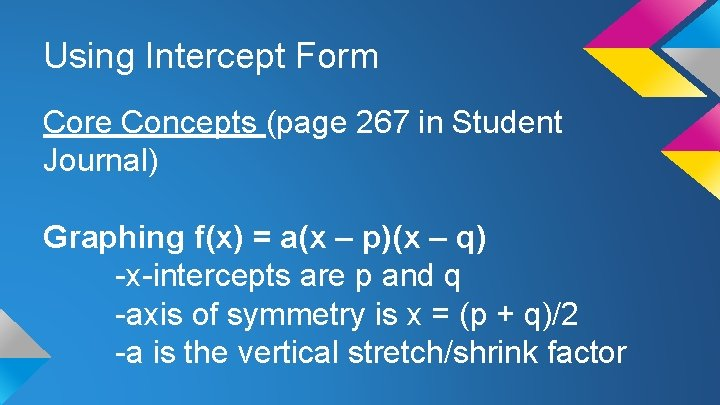Using Intercept Form Core Concepts (page 267 in Student Journal) Graphing f(x) = a(x – p)(x – q) -x-intercepts are p and q -axis of symmetry is x = (p + q)/2 -a is the vertical stretch/shrink factorUsing Intercept Form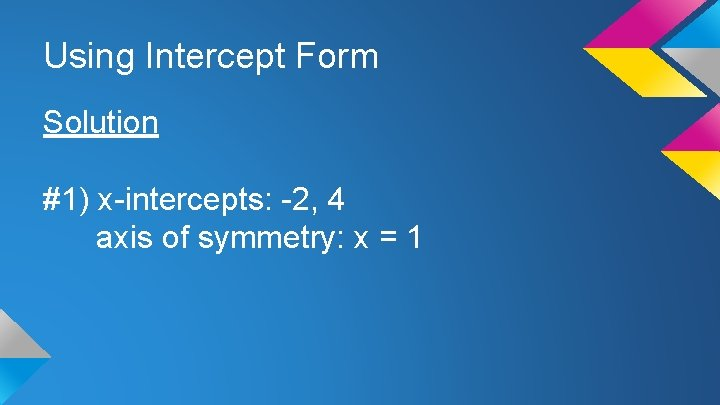Using Intercept Form Solution #1) x-intercepts: -2, 4 axis of symmetry: x = 1Using Intercept Form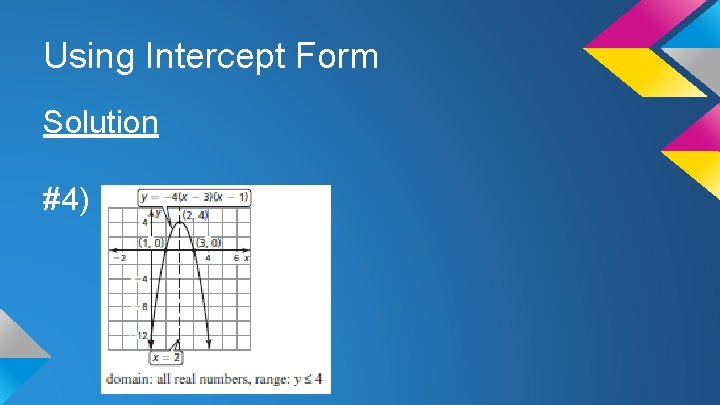Using Intercept Form Solution #4)Using Intercept FormUsing Intercept Form Solution #7) 1, -1Using Intercept FormUsing Intercept Form Solution #9)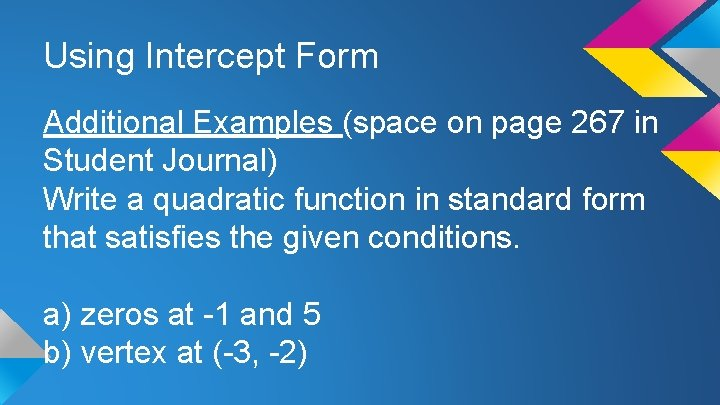Using Intercept Form Additional Examples (space on page 267 in Student Journal) Write a quadratic function in standard form that satisfies the given conditions. a) zeros at -1 and 5 b) vertex at (-3, -2)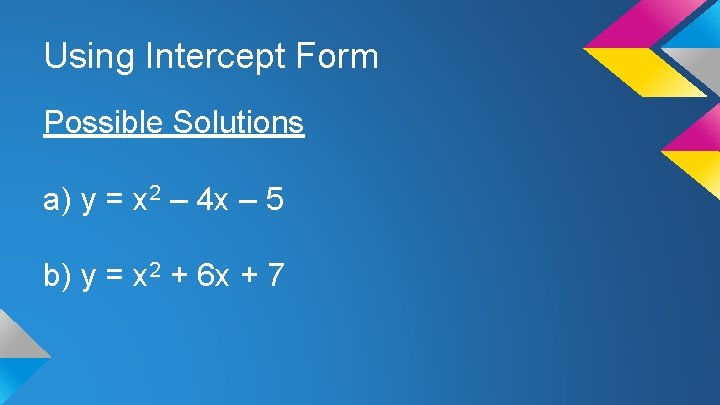Using Intercept Form Possible Solutions a) y = x 2 – 4 x – 5 b) y = x 2 + 6 x + 7Comparing Linear, Exponential, and Quadratic Functions I can choose and write functions to model data.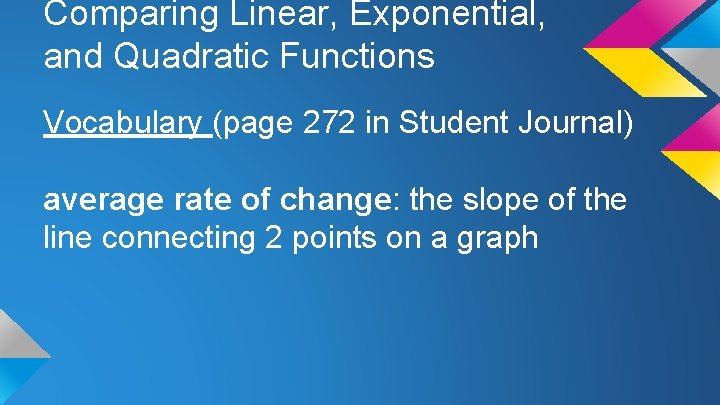Comparing Linear, Exponential, and Quadratic Functions Vocabulary (page 272 in Student Journal) average rate of change: the slope of the line connecting 2 points on a graph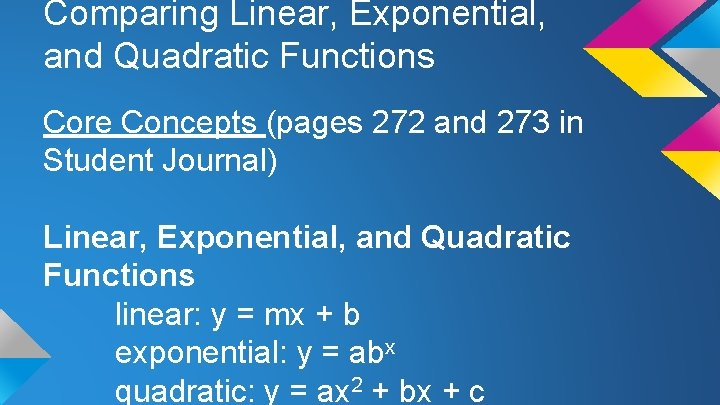Comparing Linear, Exponential, and Quadratic Functions Core Concepts (pages 272 and 273 in Student Journal) Linear, Exponential, and Quadratic Functions linear: y = mx + b exponential: y = abx quadratic: y = ax 2 + bx + cComparing Linear, Exponential, and Quadratic Functions Differences and Ratios of Functions linear: first differences of y-values are constant exponential: consecutive y-values have common ratio quadratic: second differences of yvalues are constantComparing Linear, Exponential, and Quadratic Functions Examples (pages 273 and 274 in Student Journal) Determine if the ordered pairs represent a linear, exponential or quadratic function. #1) (-3, 2), (-2, 4), (-4, 4), (-1, 8), (-5, 8) #2) (-3, 1), (-2, 2), (-1, 4), (0, 8), (2, 14)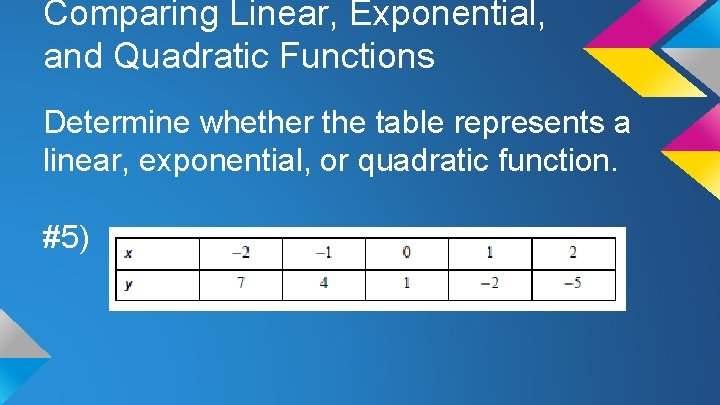Comparing Linear, Exponential, and Quadratic Functions Determine whether the table represents a linear, exponential, or quadratic function. #5)Comparing Linear, Exponential, and Quadratic Functions Solutions #5) linearComparing Linear, Exponential, and Quadratic Functions Tell whether the data represents a linear, exponential, or quadratic function. Then write the function. #8) (-2, -9), (-1, 0), (0, 3), (1, 0), (2, -9)Comparing Linear, Exponential, and Quadratic FunctionsComparing Linear, Exponential, and Quadratic Functions Additional Example (space on pages 272 and 273 in Student Journal) a) Two news website open their memberships to the public. Which site would you predict will have more members after 50 days? Why?Comparing Linear, Exponential, and Quadratic Functions Solution a) Website B, because the relationship appears to be exponential, which means the average rate of change will eventually exceed the average rate of change of website A, which is linear.